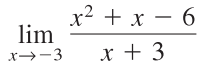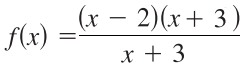# Dividing Out Technique Limits

The dividing out technique is a way to find limits by dividing out factors in the numerator and denominator. Sometimes factoring out the highest power of x will work; Other times you have to do a little leg work to identify the factors by factoring expressions.

For example, let’s say you wanted to evaluate the following limit:You can’t evaluate the limit directly, by putting in 0 for x, because that would result in an indeterminate form (0/0). You can never evaluate a limit for a function value that produces 0/0 or ∞/∞, because an indeterminate form has no meaning as a real number.

The technique works because if two functions agree except for one value (zero, in the example above), then they both have the same limit behavior at that value. Try this technique only when direct substitution fails because

## Dividing Out Technique for Limits: Examples

Example question #1: Find the limit of the following function using the dividing out technique:Solution:
Step 1: Factor the expression. We know we’re (usually) going to have to factor an expression because 99% of the time you won’t be able to divide out without factoring. If algebra isn’t your forte, there are many online factoring calculators* including this one at MathPapa which gave me the solution:

x2 + x -6 = (x – 2)(x + 3).

Replacing that expression into the function gives:Now we eliminate (x – 3):*You can usually use calculators as a short cut here because your instructor will be looking for an understanding of calculus, not factoring!

Step 2: Use direct substitution to find the limit:
“Direct substitution” can be translated to “plug in your x-value”.
From Step 1, we have f(x) = (x – 2). We’re trying to find the limit at x = -3:
f(x) = (-3 – 2) = -5.
The limit at x = -3 is -5.

Example question #2: Find the limit of the following function using the dividing out technique:Plugging in the limit at infinity results in an indeterminate form: ∞/∞

This one is a little more tricky to evaluate, because there’s nothing to factor. In this case, dividing by the highest power of x in the denominator usually helps .## References

 Kreider, D. & Lahr, D. (2002). Limits at Infinity and Infinite Limits. Retrieved April 13, 2021 from: https://math.dartmouth.edu/~m3cod/klbookLectures/204unit/infLims.pdf

CITE THIS AS:
Stephanie Glen. "Dividing Out Technique Limits" From StatisticsHowTo.com: Elementary Statistics for the rest of us! https://www.statisticshowto.com/dividing-out-technique-limits/